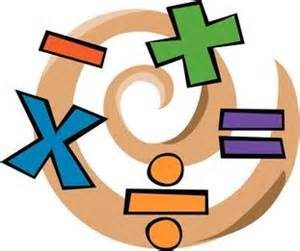# P7 Maths Homework – Revision 3Please complete the following questions in your Maths Homework jotter for Monday 22nd May. Remember to write the date, title and show all of your working and write a sentence to show your final answer, where appropriate.

Please answer the questions below in your Maths Homework jotter. Remember to write the date, a title and show all of your working.

1. Write in figures six thousand two hundred and four.
2. Multiply seven by four.
3. What is half of eight thousand five hundred?
4. Subtract two point five from ten.
5. How many millimetres are there in six and a half centimetres?
6. What is four pounds sixty-four rounded to the nearest pound?
7. Add together eighty-five and ninety-five.
8. How many minutes are there in one and a quarter hours?
9. What is the remainder when you divide forty-eight by five?
10. What is double zero point six five?
11. What is fifty-two multiplied by eight?
12. Which number is a factor of both sixty and ninety: 15, 20, 25, 40, 45
13. What is the difference between eight hundred and one hundred and seventy-nine?
14. What is twenty percent of one thousand?
15. How many vertices does a pentagonal-based pyramid have?
16. Two cereal bars cost fifty-five pence altogether. How much will it cost to buy four cereal bars?
17. Each side of a square is four and a half centimetres long. What is the perimeter of the square?
18. Write the number between one hundred and ten and one hundred and twenty whose digits add up to six.
19. What is three-eighths of fifty-six?
20. What is one minus zero point zero zero one?